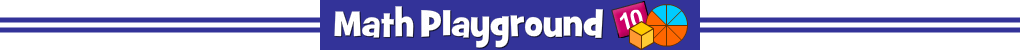There are 8 superhero masks hiding in the wall.
How many steps to uncover them all?
Practice subtraction skills. Three levels to try.
Superhero Subtraction - Learning Connections
Essential Skills
Mental Math - subtracting numbers

Common Core Connection for Grades 1 and 2
Add within 100, including adding a two-digit number and a one-digit number.
Use place value understanding and properties of operations to add and subtract.
More Math Games to Play